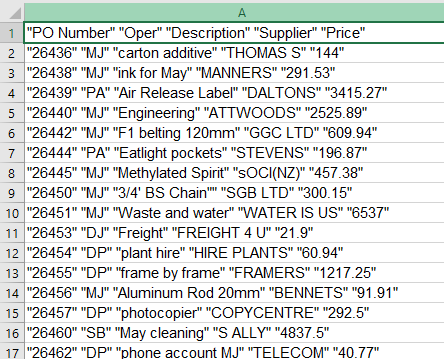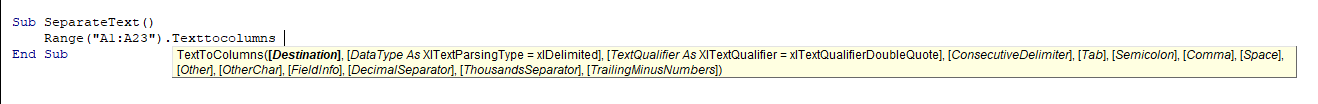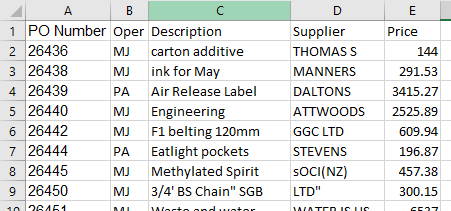# VBA Text to Columns

This tutorial will show you how to convert string of text in a single cell to multiple columns using the Range TextToColumns method in VBA

## Text to Columns

The Range.TextToColumns method in VBA is a powerful tool for cleaning up data that has been imported from text or csv files for example.

Consider the following worksheet.The data has come into Excel all in one column, and is separated by quotation marks.

You can use the Range TextToColumns method to separate this data into columns.

### TextToColumns Syntax

expression.TextToColumns (DestinationDataTypeTextQualifierConsecutiveDelimiterTabSemicolonCommaSpaceOtherOtherCharFieldInfoDecimalSeparatorThousandsSeparatorTrailingMinusNumbers)#### Expression

This is the Range of cells you wish to split – eg: Range(“A1:A23”).

All of the arguments in the TextToColumns method are optional (they have square brackets around them).

#### Destination

Where you want the result to be put – often you override the data and split it in the same location.

#### DataType

The type of text parsing you are using – it can either be xlDelimited (default if omitted), or xlFixedWidth.

#### TextQualifier

If you have quotation marks (single or double) around each field in the text that you are splitting, you need to indicate if they are single or double.

#### ConsequtiveDelimiter

This is either true or false and tells VBA to consider 2 of the same delimiters together as if it were 1 delimiter.

#### Tab

This is either True of False, the Default is False – this tells VBA that the data is delimited by a Tab.

Semicolon

This is eitherTrue of False, the Default is False – this tells VBA that the data is delimited by a Semicolon.

#### Space

This is either True of False, the Default is False – this tells VBA that the data is delimited by a Space.

#### Other

This is either True of False, the Default is False.  If you set this to True, then the next argument, OtherChar needs to be specified.

#### OtherChar

This is the character by which the text is separated (ie: ^ or | for example).

#### FieldInfo

This is an array containing information about the type of data that is being separated.  The first value in the array indicates the column number in the data, and the second value indicates the constant that you are going to use to depict the data type you require.

An example of for 5 columns with data types of text, numbers and dates could be:

Array(Array(1, xlTextFormat), Array(2, xlTextFormat), Array(3, xlGeneralFormat), Array(4, xlGeneralFormat), Array(5, xlMDYFormat))

Another way of setting this out is:

Array(Array(1, 2), Array(2, 2), Array(3, 1), Array(4, 1), Array(5, 3))

The numbers in the second column are the values of the constants where the constant xlTextFormat has a value of 2, the xlGeneralFormat (default) has a value of 1 and the xlMDYFormat has a value of 3.

#### DecimalSeparator

You can specify the decimal separator that VBA must use to if there are numbers in the data. If omitted, it will use the system setting, which is usually a period.

#### ThousandsSeparator

You can specify the thousands separator that VBA must use to if there are numbers in the data. If omitted, it will use the system setting, which is usually a comma.

#### TrailingMinusNumbers

This argument is largely for compatibility for data that is generated from older systems where a minus sign was often after the number and not before. You should set this to True if negative numbers have the minus sign behind them.  The Default is False.

### Converting Text to Columns

The following procedure will convert the Excel data above into columns.

``````Sub TextToCol1()
Range("A1:A25").TextToColumns _
Destination:=Range("A1:A25"),
DataType:=xlDelimited, _
TextQualifier:=xlDoubleQuote, _
ConsecutiveDelimiter:=True, _
Tab:=False, _
Semicolon:=False, _
Comma:=False,
Space:=True, _
Other:=False, _
FieldInfo:=Array(Array(1, 1), Array(2, 1), Array(3, 1), Array(4, 1), Array(5, 1)), _
DecimalSeparator:="." , _
ThousandsSeparator:=",", _
TrailingMinusNumbers:=True
End Sub``````

In the above procedure we have filled in all of the parameters.  However, many of the parameters are set to false or to the default setting and are not necessary. A cleaner version of the above procedure is set out below. You need to use the parameter names to indicate which parameters we are using.

``````Sub TextToCol2()
Range("A1:A25").TextToColumns _
DataType:=xlDelimited, _
TextQualifier:=xlDoubleQuote, _
ConsecutiveDelimiter:=True, _
Space:=True,
End Sub``````

There are only 4 parameters that are actually required – the data is delimited by a double quote, you want consecutive quotes treated as one and the data is separated by a space!

For an even speedier line of code, we could omit the parameter names, but then we would need to put in commas to save the place of the parameter. You only need to put information as far as the last parameter you are using – in this case the Space that separates the data which is the 8th parameter.

``````Sub TextToCol3()
Range("A1:A25").TextToColumns , xlDelimited, xlDoubleQuote, True, , , , True
End Sub``````

Once you run the any of the procedures above, the data will be separated as per the graphic below.Stop searching for VBA code online. Learn more about AutoMacro - A VBA Code Builder that allows beginners to code procedures from scratch with minimal coding knowledge and with many time-saving features for all users!